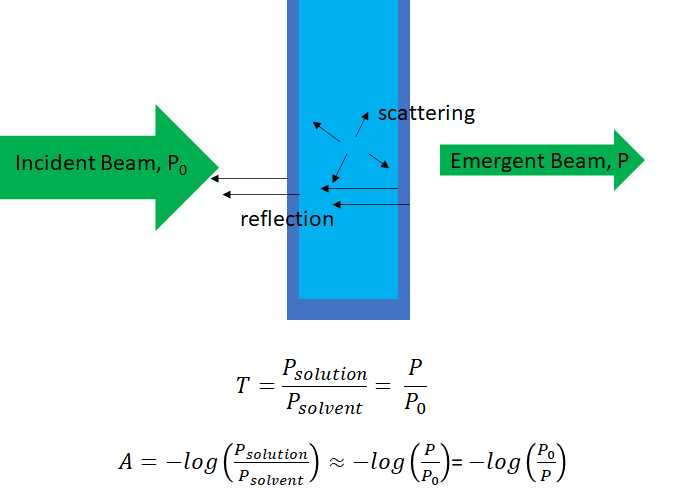# 1: Measurement of Transmittance and Absorbance

$$\newcommand{\vecs}{\overset { \rightharpoonup} {\mathbf{#1}} }$$ $$\newcommand{\vecd}{\overset{-\!-\!\rightharpoonup}{\vphantom{a}\smash {#1}}}$$$$\newcommand{\id}{\mathrm{id}}$$ $$\newcommand{\Span}{\mathrm{span}}$$ $$\newcommand{\kernel}{\mathrm{null}\,}$$ $$\newcommand{\range}{\mathrm{range}\,}$$ $$\newcommand{\RealPart}{\mathrm{Re}}$$ $$\newcommand{\ImaginaryPart}{\mathrm{Im}}$$ $$\newcommand{\Argument}{\mathrm{Arg}}$$ $$\newcommand{\norm}{\| #1 \|}$$ $$\newcommand{\inner}{\langle #1, #2 \rangle}$$ $$\newcommand{\Span}{\mathrm{span}}$$ $$\newcommand{\id}{\mathrm{id}}$$ $$\newcommand{\Span}{\mathrm{span}}$$ $$\newcommand{\kernel}{\mathrm{null}\,}$$ $$\newcommand{\range}{\mathrm{range}\,}$$ $$\newcommand{\RealPart}{\mathrm{Re}}$$ $$\newcommand{\ImaginaryPart}{\mathrm{Im}}$$ $$\newcommand{\Argument}{\mathrm{Arg}}$$ $$\newcommand{\norm}{\| #1 \|}$$ $$\newcommand{\inner}{\langle #1, #2 \rangle}$$ $$\newcommand{\Span}{\mathrm{span}}$$$$\newcommand{\AA}{\unicode[.8,0]{x212B}}$$

In absorption spectroscopy a beam of light is incident on a sample and most of the light passes through the sample.  The intensity of the light emerging from the sample is attenuated by reflections losses at each of four interfaces where the refractive index of the media change (air/container wall, container wall/solution, solution/container wall and container wall/air), possibly attenuated by particles scattering the light in the sample, and, most importantly, by the absorption of the light by the sample.   In order for the sample to absorb the light two conditions need be met; (1) there must be a mechanism by which a component of the sample can interact with the electric or magnetic field components of the light and (2) the wavelength or energy of the light must be resonant (match) the difference in energy between two of the quantized energy levels of the absorbing component.Figure $$\PageIndex {1}$$: An illustration of the passage of light through a sample (light blue) and the container (dark blue) also showing reflections losses at the four interfaces where the refractive index changes and light loses due to scattering.

The transmittance, T, is simply the fraction of light intensity passing through the sample and the absorbance, A, is the - log10 of the intensity of the light passing though the solvent relative to the intensity of light passing though the sample.  For a non-absorbing solvent A = - log(P0/P)

1: Measurement of Transmittance and Absorbance is shared under a not declared license and was authored, remixed, and/or curated by LibreTexts.